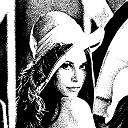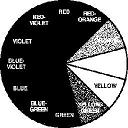Digital Image Processing
Basic effects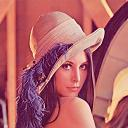<- BACK

 Conversion from colors to gray level

Simple
The simplest and faster way to grayscale.
Average of Red, Green and Blue chanels.

G = (R+G+B)/3

Languages : C & MatLab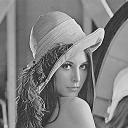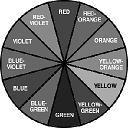YUV
Using the 'Y' chanel, the luma component
(the brightness) of the picture
This methode gives more contrast than the average.

Y = (0,299*R) + (0,587*V) + (0,114*B)

Languages : C & MatLab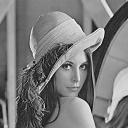Conversion from colors to black and white(Based on a grayscale picture)

Simple
Threshold at half a byte (127).
The simplest and faster way to make a black and white picture.

If IN>127 -> OUT=255
Else -> OUT=0

Languages : C & MatLab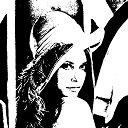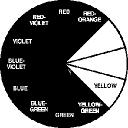Random
Threshold at half a byte adding an error [108 , 148]
More useful for printing, but unusable for searching algorithms.

If IN> (107+Alea[0,40]) -> OUT=255
Else -> OUT=0

Language : C